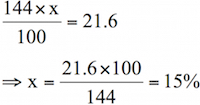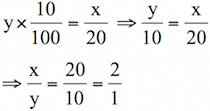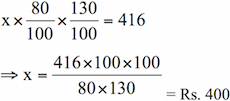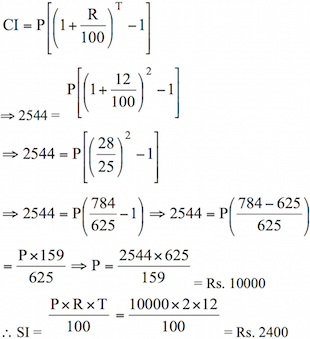# Sample Aptitude Questions of Dell

1. The list price of an article is Rs. 160 and a customer buys it for Rs. 122.40 after two successive discounts. If the first discount is 10%, then second discount is
1. 12%
2. 10%
3. 14%
4. 15%
SP after a discount of 10%
= (160*90) / 100 = Rs. 144
Second discount
= 144 – 122.40 = Rs. 21.6
If the second discount be x% then1. In a school, 10% of number of girls is equal to 1/20 of number of boys. Ratio between the 'number of boys to number of girls is
1. 1:2
2. 2:1
3. 1:4
4. 4:1
LIf boys = x and girls = y, then2. The ratio of 252.5 : 53 is same as
1. 5 : 3
2. 5 : 6
3. 1 : 25
4. 25 : 1
252.5 : 53 = (52)2.5 : 53
= 55 : 53 = 52 : 1 = 25 : 1
3. If a, b, c, d, e are five consecutive odd numbers, their average is
1. 5(a + 4)
2. abde/5
3. 5(a + b + c + d + e)
4. a + 4
b = a + 2
c = b + 2 = a + 4
d = c + 2 = a + 6
e = d + 2 = a + 8
Therefore, Required average = (a + a + 2 + a + 4 + a + 6 + a + 8)/5
= a + 4
4. The average of 20 numbers is 15 and the average of first five is 12. The average of the rest is
1. 16
2. 15
3. 14
4. 13
If the average of remaining numbers be x, then
20 × 15 = 5 × 12 + 15x
⇒ 300 = 60 + 15x
⇒ 15x = 300 – 60 = 240
=> x = 240/15 = 16
5. A tradesman sold an article at a loss of 20%. If the selling price had been increased by Rs. 100, there would have been a gain of 5%. The cost price of the article (in Rs.) was
1. 100
2. 200
3. 400
4. 500
C. P. of article = Rs. X
∴ First SP = 80x/100 = Rs. 4x/5
Case II⇒ 5x = 2000 ⇒ x = 2000/5 = Rs. 400
6. The price of an article is first decreased by20% and then increased by 30%. If the resulting price is Rs. 416, the original price of the article is
1. Rs. 350
2. Rs. 405
3. Rs. 400
4. Rs. 450
If the original price of article be Rs. X, then7. A man performs 2/15 of the total journey by train 9/20 by bus and the remaining 10 km on foot. His total journey in km is
1. 15.6
2. 24
3. 16.4
4. 12.8
If the total journey be x km, then
2x/15+9x/20+10=x
=>x-2x/15-9x/20=10
=> 60x-8x-27x/60=10
=> 25x/60=10
=> X=60*10/25=24km
8. By walking at 3/4 of his usual speed, a man reaches his office 20 minutes later than usual. His usual time is
1. 30 min
2. 75 min
3. 90 min.
4. 60 min.